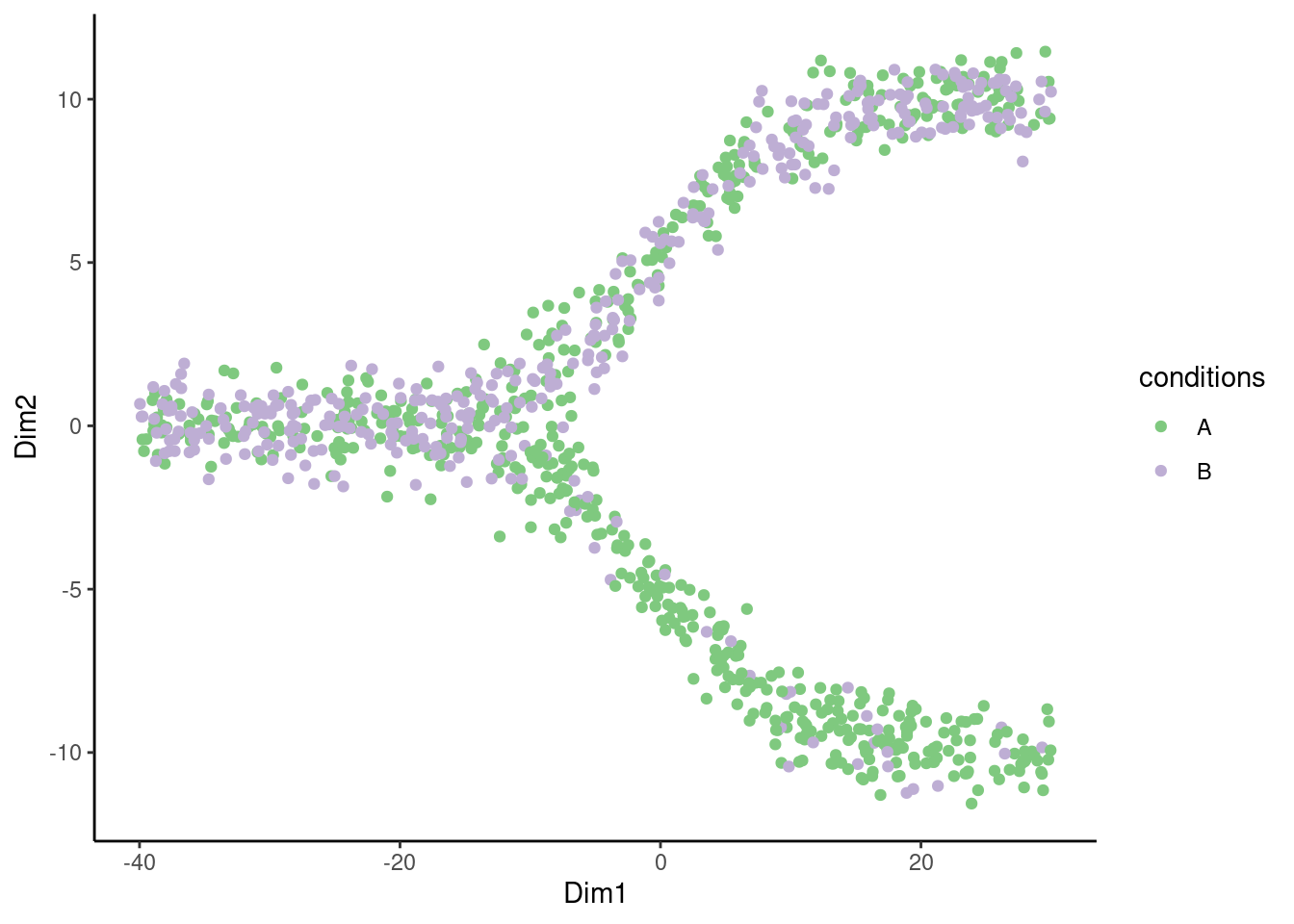# Initial pre-processing

## Generating a synthetic dataset

We will use a synthetic dataset to illustrate the functionalities of the condiments package. We start directly with a dataset where the following steps are assumed to have been run:

• Obtaining count matrices for each setting (i.e. each condition).
• Integration and normalization between the conditions.
• Reduced Dimension Estimations
• (Clustering)
``````# For analysis
library(condiments)
library(slingshot)
# For data manipulation
library(dplyr)
library(tidyr)
# For visualization
library(ggplot2)
library(RColorBrewer)
library(viridis)
set.seed(2071)
theme_set(theme_classic())``````
``````data("toy_dataset", package = "condiments")
df <- toy_dataset\$sd``````

As such, we start with a matrix `df` of metadata for the cells: coordinates in a reduced dimension space `(Dim1, Dim2)`, a vector of conditions assignments `conditions` (A or B) and a lineage assignment.

## Vizualisation

We can first plot the cells on the reduced dimensions

``````p <- ggplot(df, aes(x = Dim1, y = Dim2, col = conditions)) +
geom_point() +
scale_color_brewer(type = "qual")
p``````We can also visualize the underlying skeleton structure of the two conditions.

``````p <- ggplot(df, aes(x = Dim1, y = Dim2, col = conditions)) +
geom_point(alpha = .5) +
geom_point(data = toy_dataset\$mst, size = 2) +
geom_path(data = toy_dataset\$mst, aes(group = lineages), size = 1.5) +
scale_color_brewer(type = "qual") +
facet_wrap(~conditions) +
guides(col = FALSE)
p``````